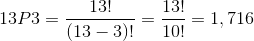# Algebra II : Algebra II

## Example Questions

### Example Question #41 : Calculating Probability

Hector has a shelf of books. He wants to choose a random book to read. If there are 24 books on the shelf and he has an equal chance of choosing any particular one, what is the probability that he picks the third book from the left?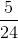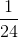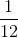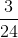Explanation:

If Hector has a shelf of books. He wants to choose a random book to read. If there are 24 books on the shelf and he has an equal chance of choosing any particular one, what is the probability that he picks the third book from the left?

This is a wordy problem, but if we cut through to what it's asking it is fairly simple.

We need to find the probability of an event.

The event is randomly choosing a book from a book shelf.

There are 24 books total, and we need to pick 1.

So, our probability will be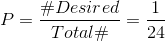### Example Question #41 : Calculating Probability

Julie tosses a fair coin twice, and then rolls a six-sided die.  What is the probability that she will flip two tails, and roll a number other than four?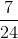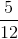Explanation:

Each event is independent of another.

A fair coin has either heads or tails, which means the probability of her rolling heads or tails will be.

The probability of her rolling two tails is: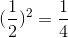In a six sided dice, the odds of rolling any particular number is one-sixth.

If Julie was to roll a number other than four, this means that she has five out of six possible rolls that she can land on.

The probability of rolling a number other than four is: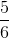Multiply the probabilities.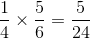The answer is:### Example Question #1 : Permutations

Find the Computing Permutation.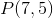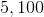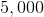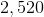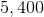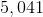Explanation: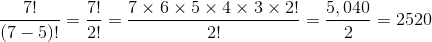### Example Question #1 : How To Find The Number Of Integers Between Two Other Integers

An ice cream vendor sells five different flavors of ice cream.

In how many ways can you choose three scoops of different ice cream flavors if order matters?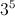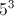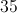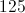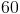Explanation:

There are five ways to choose the first scoop, then four ways to choose the second scoop, and finally three ways to choose the third scoop:

5 * 4 * 3 = 60

### Example Question #1 : Permutations

There are 5 men and 4 women competing for an executive body consisting of :

1. President
2. Vice President
3. Secretary
4. Treasurer

It is required that 2 women and 2 men must be selected

How many ways the executive body can be formed?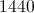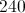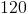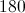Explanation:

2 men can be selected: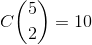2 women can be selected out of 4 women: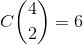Finally, after the selection process, these men and women can fill the executive body in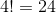ways.

This gives us a total of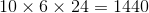### Example Question #2 : Permutations

How many ways can a three committee board select the president, vice president and treasurer from a group of 15 people?

None of the above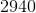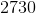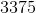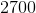Explanation:

In this problem, order is important because once someone is chosen as a position they can not be chosen again, and once a position is filled, no one else can fill that in mind.

The presidential spot has a possibility of 15 choices, then 14 choices for vice president and 13 for the treasurer.

So: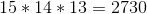### Example Question #3 : Permutations

How many ways can you re-arrange the letters of the word JUBILEE?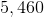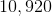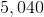Explanation:

There are 7 letters in the word jubilee, so initially we can calculate that there are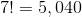ways to re-arrange those letters. However, The letter e appears twice, so we're double counting. Divide by 2 factorial (2) to get.

### Example Question #4 : Permutations

How many ways can you re-arrange the letters of the word BANANA?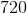Explanation:

At first, it makes sense that there are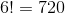ways to re-arrange these letters. However, the letter A appears 3 times and the letter N appears twice, so divide first by 3 factorial and then 2 factorial: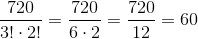### Example Question #5 : Permutations

In a class of 24 students, how many distinct groups of 4 can be formed?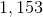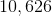Explanation:

To solve, evaluate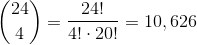### Example Question #1 : Permutations

13 rubber ducks are competing in a race. How many different arrangements of first, second, and third place are possible?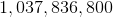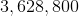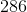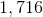There are 3 winners out of the total set of 13. That means we're calculating